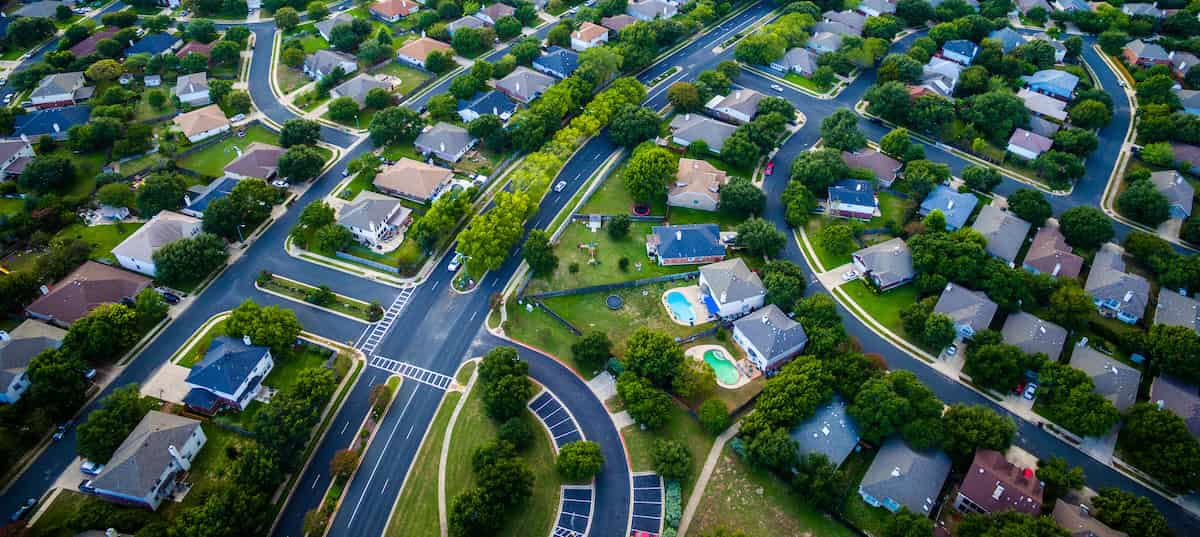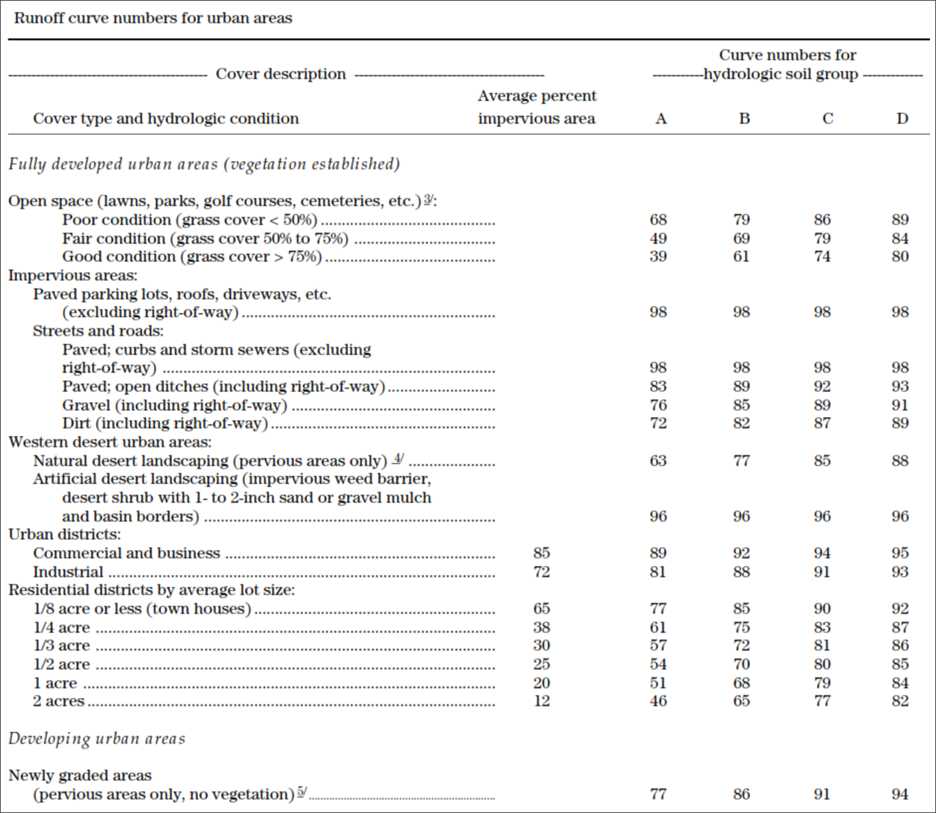1. Home
2. Knowledge Base
3. GeoHECHMS
4. Curve Numbers for Urban Impervious Areas

# Curve Numbers for Urban Impervious Areas

Several factors, such as the percentage of impervious area and the means of conveying runoff from impervious areas to the drainage system, should be considered in computing curve numbers for urban areas. For example, do the impervious areas connect directly to the drainage system, or do they outlet onto lawns or other pervious areas where infiltration can occur?## Connected Impervious Areas

An impervious area is considered connected if runoff from it flows directly into the drainage system. It is also considered connected if runoff from it occurs as a concentrated shallow flow that runs over a pervious area and then into the drainage system.Urban curve numbers were developed for typical land use relationships based on specific assumed percentages of impervious area. These CN values were developed on the assumptions that:

• pervious urban areas are equivalent to pasture in good hydrologic condition, and
• impervious areas have a CN of 98 and are directly connected to the drainage system

Some assumed percentages of impervious area are shown in the below table.If all the impervious area is directly connected to the drainage system, but the impervious area percentages or the pervious land use assumptions in the above table are not applicable, then use the below figure to compute a composite CN. For example, the above table gives a CN of 70 for a 1/2-acre lot in a hydrologic soil group B, with an assumed impervious area of 25 percent. However, if the lot has a 20 percent impervious area and a pervious area CN of 61, the composite CN obtained from the below figure is 68. The CN difference between 70 and 68 reflects the difference in percent impervious area.## Unconnected Impervious Areas

Runoff from these areas is spread over a pervious area as sheet flow.To determine CN when all or part of the impervious area is not directly connected to the drainage system, then:

• use the figure 2 if the total impervious area is less than 30 percent or
• use the figure 1 if the total impervious area is equal to or greater than 30 percent because the absorptive capacity of the remaining pervious areas will not significantly affect runoff

When impervious area is less than 30 percent, obtain the composite CN by entering the right half of the below figure with the percentage of total impervious area and the ratio of total unconnected impervious area to total impervious area. Then move left to the appropriate pervious CN and read down to find the composite CN. For example, for a 1/2-acre lot with 20 percent total impervious area (75 percent of which is unconnected) and pervious CN of 61, the composite CN from the below figure is 66. If all the impervious area is connected, the resulting CN (from the above figure) would be 68.## Automated Curve Number Computation

In GeoHECHMS, the Compute CN command can be used to automatically compute the curve number (CN) to determine how much runoff occurs based upon the Land Use (or Land Cover) and the underlying Hydrologic Soil Type. To learn more about the Compute CN command, refer to this article in our knowledge base.